# Problem: 1. An object moves clockwise with decreasing speed around an oval track. The velocity vectors at points G and H are shown. a. In the space at right, copy the velocity vectors  and . From these vectors, determine the change in velocity vector, .b. If point H were chosen to lie closer to point G, describe how  would change (direction and magnitude). c. Describe how you would determine the acceleration (direction and magnitude) at point G. In the space at right, indicate the direction of the acceleration of the object at point G. d. Copy  and  (placed "tail-to-tail") in the space at right. How does the angle between the acceleration and velocity vectors compare to 90°? (i.e., Is the angle greater than, less than, or equal to 90°?) e. Generalize your results above and from tutorial to answer the following question: For an object moving along a curved trajectory, how does the angle between the acceleration and velocity vectors compare to 90° if the object moves with (i) constant speed, (ii) increasing speed, and (in) decreasing speed?

###### FREE Expert Solution

a.

The resultant vector, Δv, from vectors, vG and vH is,

$\mathbf{∆}\stackrel{\mathbf{⇀}}{\mathbf{v}}\mathbf{=}{\stackrel{\mathbf{⇀}}{\mathbf{v}}}_{\mathbf{H}}\mathbf{-}{\stackrel{\mathbf{⇀}}{\mathbf{v}}}_{\mathbf{G}}$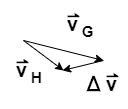99% (423 ratings)###### Problem Details

1. An object moves clockwise with decreasing speed around an oval track. The velocity vectors at points G and H are shown.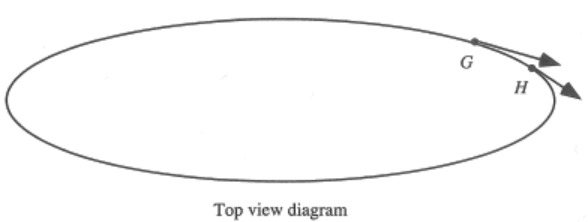a. In the space at right, copy the velocity vectors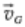and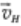. From these vectors, determine the change in velocity vector,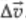.

b. If point H were chosen to lie closer to point G, describe howwould change (direction and magnitude).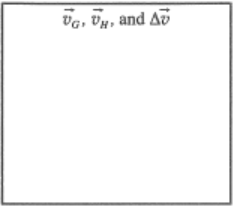c. Describe how you would determine the acceleration (direction and magnitude) at point G. In the space at right, indicate the direction of the acceleration of the object at point G.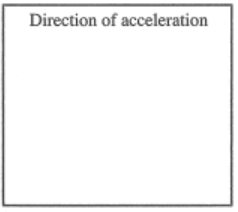d. Copyand(placed "tail-to-tail") in the space at right. How does the angle between the acceleration and velocity vectors compare to 90°? (i.e., Is the angle greater than, less than, or equal to 90°?)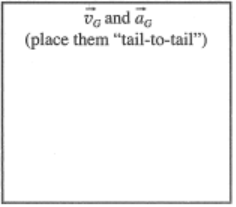e. Generalize your results above and from tutorial to answer the following question:

For an object moving along a curved trajectory, how does the angle between the acceleration and velocity vectors compare to 90° if the object moves with (i) constant speed, (ii) increasing speed, and (in) decreasing speed?